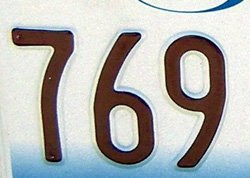## Thursday, June 18, 2009

### 769

769 is a prime number.

769 is the total number of digits of all binary numbers of length 1 to 7.

769 is 30001 in base 4.

769 has a representation as a sum of two squares: 769 = 122 + 252.

769 is the hypotenuse of a primitive Pythagorean triple: 7692 = 4812 + 6002.769 Tatjana is an asteroid orbiting the sun.

Source: What’s Special About This Number?